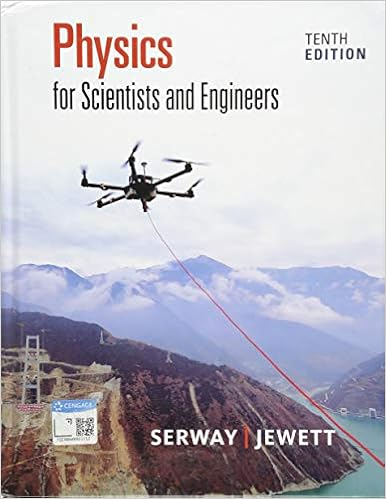# Physics 2125 Exp 2 - Experiment 2 Vector Addition Physics...

• Lab Report
• 6
• 86% (14) 12 out of 14 people found this document helpful

This preview shows page 1 - 4 out of 6 pages.

##### We have textbook solutions for you!
The document you are viewing contains questions related to this textbook.The document you are viewing contains questions related to this textbook.
Chapter 7 / Exercise 7.3
Physics for Scientists and Engineers
Jewett/SerwayExpert Verified
Experiment 2: Vector Addition Physics 2125 (16008) HCC-Scarcella Science & Technology September 2, 2016 Objective
##### We have textbook solutions for you!
The document you are viewing contains questions related to this textbook.The document you are viewing contains questions related to this textbook.
Chapter 7 / Exercise 7.3
Physics for Scientists and Engineers
Jewett/SerwayExpert Verified
The first objective of this experiment was to calculate the number of forces acting on a body and then calculate the resultant of the same forces by drawing a vector diagram to scale to find its magnitude and direction. Once this was done, we calculated the resultant using analytical methods, most notably the law of cosine for magnitude and the law of sine in order to obtain the direction in one instance. The components method is also used. Equipment 1. Force table 2. Weight holders (#4). 3. Pulleys (#4). 4. Slotted weights 5. Strings for suspending the masses 6. A ring 7. A metal pin 8. A protractor 9. Graph paper. Theory and Equations Vectors such as force, displacement, velocity are physical quantities with both magnitude and direction. In contrast, a scalar value is a physical quantity that only has magnitude. Examples of include speed, temperature and distance. A vector A can be written as the sum of two vectors A x and A y and can be drawn along the x and y-axis respectively. A x and A y are called the components of vector A and are given by : A x = A cosα (Equation 1) A y = A sinα α (≤ 90 0 ) is the angle vector A makes with the x-axis. (Equation 2) To find the resultant, R , of the vectors A, B, C etc we much follow the following steps: 1. Obtain the A x, B x, C x ... and A y, B y, C y .... components of each vector using the above two equation. We must keep in mind that the Vector value can be positive or negative depending in its direction. 2. Once we have the above values we write them down as: R x = A x + B x + C x + … R y = A y + B y + C y + … 3. The magnitude of R can now be calculated using the equation [R x 2 + R y 2 ] ½ and the direction of R can be calculated using the equation θ = tan -1 [R y / R x ] where θ is the angle between R and x axis.
If θ > 0 then R is either in the 1 st or 3 rd quadrant
•••